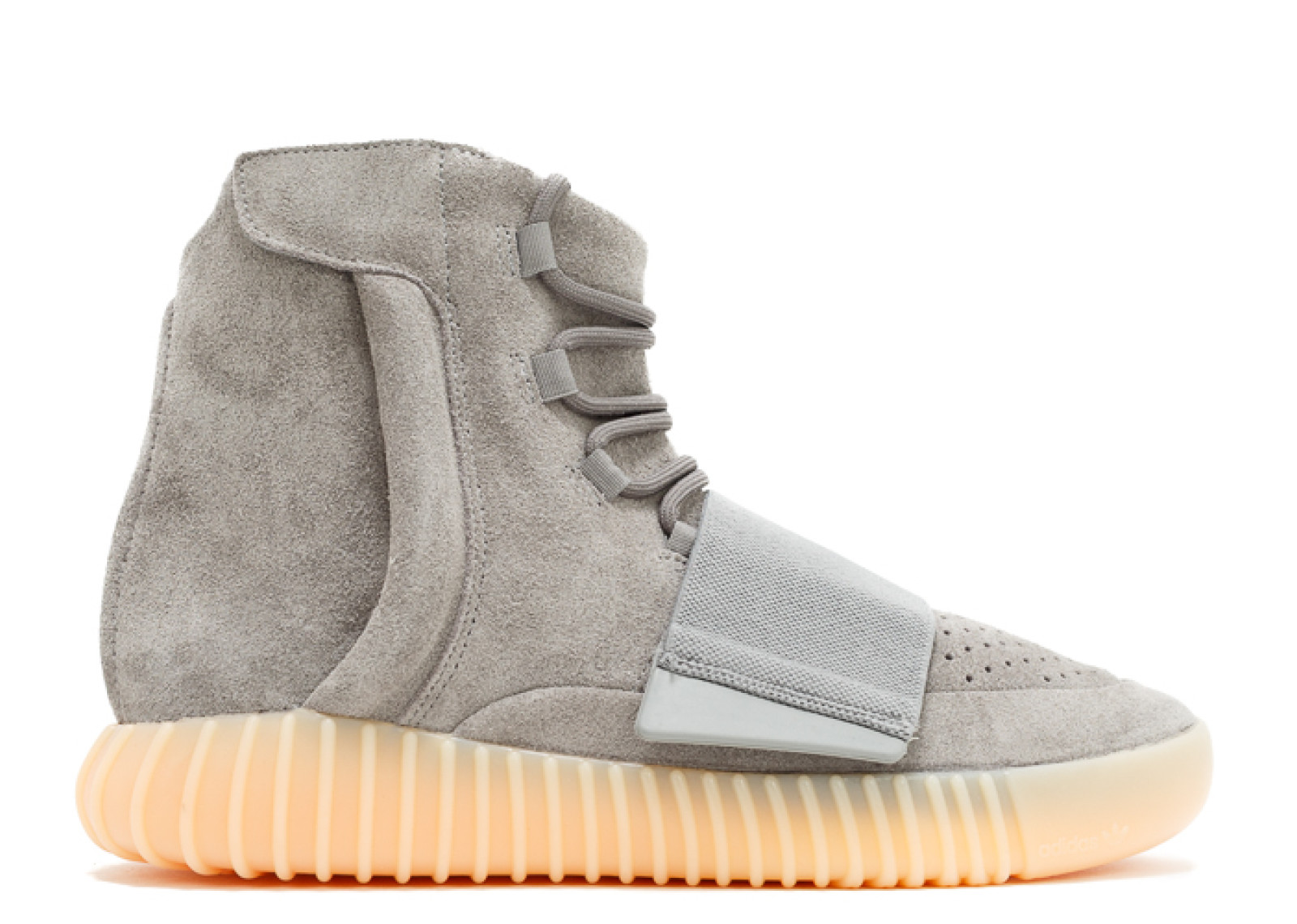All Categories
US\$

# Adidas Yeezy Boost 750 Gum Grey/Glow Sole 2016

US\$ 185.00
Quality Control:
QC by FKZ team (Get shipped faster) QC by myself (1-3 days prepation time)
US10.5=UK10=EU442/3=28.5
US\$ 185.00
US10=UK9.5=EU44=28CM
US\$ 185.00
US11.5=UK11=EU46=29.5CM
US\$ 185.00
US11=UK10.5=EU451/3=29CM
US\$ 185.00
US12=UK11.5=EU46.7=30CM
US\$ 185.00
US7.5=UK7=EU402/3=25.5CM
US\$ 185.00
US7=UK6.5=EU40=25CM
US\$ 185.00
US8.5=UK8=EU42=26.5CM
US\$ 185.00
US8=UK7.5=EU411/3=26CM
US\$ 185.00
US9.5=UK9=EU431/3=27.5CM
US\$ 185.00
US9=UK8.5=EU422/3=27CM
US\$ 185.00
Quantity: 0 , Subtotal 0